Saturday, July 11, 2020
Home > CBSE Class 12 > NCERT Solutions for Class 12 Maths Vector Algebra Miscellaneous Exercise

# NCERT Solutions for Class 12 Maths Vector Algebra Miscellaneous Exercise# NCERT Solutions for Class 12 Maths Vector Algebra Miscellaneous Exercise

Hi Students, Welcome to Amans Maths Blogs (AMB). In this post, you will get the NCERT Solutions for Class 12 Maths Vector Algebra Miscellaneous Exercise.

CBSE Class 12th is an important school class in your life as you take some serious decision about your career. And out of all subjects, Maths is an important and core subjects. So CBSE NCERT Solutions for Class 12th Maths is major role in your exam preparation as it has detailed chapter wise solutions for all exercise. This NCERT Solutions can be downloaded in PDF file. The downloading link is given at last.

NCERT Solutions for class 12 is highly recommended by the experienced teacher for students who are going to appear in CBSE Class 12 and JEE Mains and Advanced and NEET level exams. Here You will get NCERT Solutions for Class 12 Maths Vector Algebra Miscellaneous Exercise of all questions given in NCERT textbooks of class 12 in details with step by step process.

NCERT Solutions for Class 12 Maths are not only the solutions of Maths exercise but it builds your foundation of other important subjects. Getting knowledge of depth concept of CBSE Class 12th Maths like Algebra, Calculus, Trigonometry, Coordinate Geometry help you to understand the concept of Physics and Physical Chemistry.

As we know that all the schools affiliated from CBSE follow the NCERT books for all subjects. You can check the CBSE NCERT Syllabus. Thus, NCERT Solutions helps the students to solve the exercise questions as given in NCERT Books

The PDF books of NCERT Solutions for Class 12 are the first step towards the learning and understanding the each sections of Maths, Physics, Chemistry, Biology as it all help in engineering medical entrance exams. To solve it, you just need to click on download links of NCERT solutions for class 12.

## NCERT Solutions for Class 12 Maths Vector Algebra Miscellaneous Exercise

NCERT Solutions Class 12 Math Vector Algebra Miscellaneous Exercise Ques No 1

Write down a unit vector in XY-plane, making an angle of 30° with the positive direction of x-axis.

NCERT Solutions: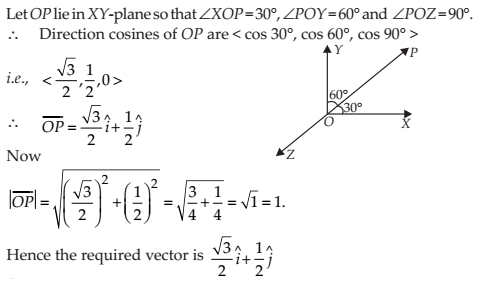NCERT Solutions Class 12 Math Vector Algebra Miscellaneous Exercise Ques No 2

Find the scalar components and magnitude of the vector joining the points P(x1, y1, z1) and Q (x2, y2, z2).

NCERT Solutions:NCERT Solutions Class 12 Math Vector Algebra Miscellaneous Exercise Ques No 3

A girl walks 4 km towards west, then she walks 3 km in a direction 30° east of north and stops. Determine the girl’s displacement from her initial point of departure..

NCERT Solutions: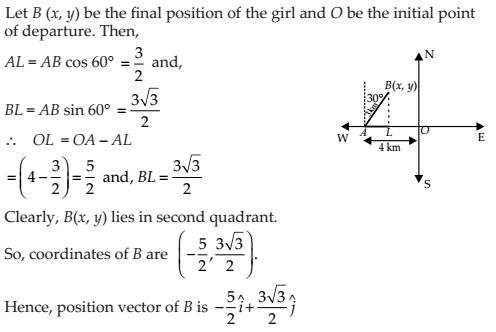NCERT Solutions Class 12 Math Vector Algebra Miscellaneous Exercise Ques No 4

If a = b + c, then is it true that | a | = | b | + | c |? Justify your answer.

NCERT Solutions:NCERT Solutions Class 12 Math Vector Algebra Miscellaneous Exercise Ques No 5

Find the value of x for which x(i + j + k) is a unit vector.

NCERT Solutions: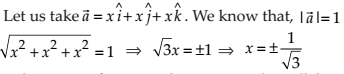NCERT Solutions Class 12 Math Vector Algebra Miscellaneous Exercise Ques No 6

Find a vector of magnitude 5 units, and parallel to the resultant of the vectors a = 2i + 3jk and b = i – 2j + k.

NCERT Solutions: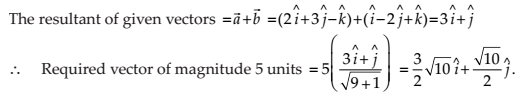NCERT Solutions Class 12 Math Vector Algebra Miscellaneous Exercise Ques No 7

If a = i + j + k, b = 2ij + 3k and c = i – 2j + k, find a unit vector parallel to the vector 2ab + 3c.

NCERT Solutions:NCERT Solutions Class 12 Math Vector Algebra Miscellaneous Exercise Ques No 8

Show that the points A(1, – 2, – 8), B (5, 0, – 2) and C (11, 3, 7) are collinear, and find the ratio in which B divides AC.

NCERT Solutions: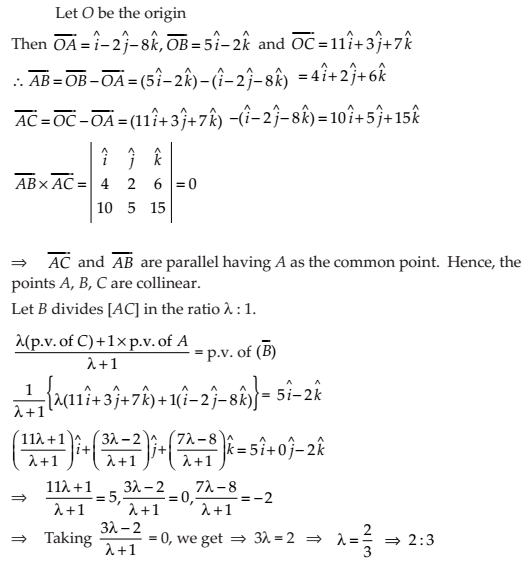NCERT Solutions Class 12 Math Vector Algebra Miscellaneous Exercise Ques No 9

Find the position vector of a point R which divides the line joining two points P and Q whose position vectors are (2a + b) and (a – 3b) externally in the ratio 1 : 2. Also, show that P is the mid point of the line segment RQ.

NCERT Solutions: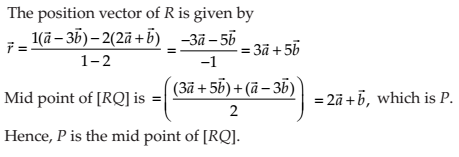NCERT Solutions Class 12 Math Vector Algebra Miscellaneous Exercise Ques No 10

The two adjacent sides of a parallelogram are 2i − 4j + 5k and i − 2j − 3k. Find the unit vector parallel to its diagonal. Also, find its area.

NCERT Solutions:NCERT Solutions Class 12 Math Vector Algebra Miscellaneous Exercise Ques No 11

Show that the direction cosines of a vector equally inclined to the axes OX, OY and OZ are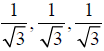.

NCERT Solutions: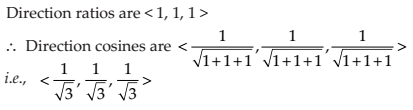NCERT Solutions Class 12 Math Vector Algebra Miscellaneous Exercise Ques No 12

Let a = i + 4j + 2k, b = 3i – 2j + 7k and c = 2ij + 4k. Find a vector d which is perpendicular to both a and b, and c.d = 15.

NCERT Solutions:NCERT Solutions Class 12 Math Vector Algebra Miscellaneous Exercise Ques No 13

The scalar product of the vector i + j + k with a unit vector along the sum of vectors 2i + 4j − 5k and λi + 2j + 3k is equal to one. Find the value of λ.

NCERT Solutions:NCERT Solutions Class 12 Math Vector Algebra Miscellaneous Exercise Ques No 14

If a, b, c are mutually perpendicular vectors of equal magnitudes, show that the vector a + b + c is equally inclined to a, b and c.

NCERT Solutions: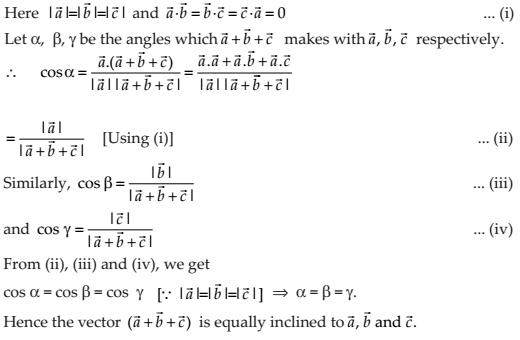NCERT Solutions Class 12 Math Vector Algebra Miscellaneous Exercise Ques No 15

Prove that (a + b).(a + b) = |a|2 + |b|2, if and only if a, b are perpendicular, given a0 and b0.

NCERT Solutions:Choose the correct answer in Exercises 16 to 19.

NCERT Solutions Class 12 Math Vector Algebra Miscellaneous Exercise Ques No 16

If θ is the angle between two vectors a and b, then ab ≥ 0 only when

(A) 0 < θ < π/2

(B) 0 ≤ θ ≤ π/2

(C) 0 < θ < π

(D) 0 ≤ θ ≤ π/2

NCERT Solutions:

(B) ab ≥ 0 or, |a||b|cosθ ≥ 0 or  cosθ ≥ 0 or 0 ≤ θ ≤ π/2

NCERT Solutions Class 12 Math Vector Algebra Miscellaneous Exercise Ques No 17

Let a and b be two unit vectors and θ is the angle between them. Then a + b is a unit vector if

(A) θ = π/4

(B) θ = π/3

(C) θ = π/2

(D) θ = 2π/3

NCERT Solutions:NCERT Solutions Class 12 Math Vector Algebra Miscellaneous Exercise Ques No 18

The value of i.(j × k) + j⋅(i × k) + k⋅(i × j) is

(A) 0

(B) -1

(C) 1

(D) 3

NCERT Solutions:

(C) i.(j × k) + j⋅(i × k) + k⋅(i × j) = i.i + j⋅(-j) + kk = 1 – 1 + 1 = 1

NCERT Solutions Class 12 Math Vector Algebra Miscellaneous Exercise Ques No 19

If θ is the angle between any two vectors a and b, then | ab | = | a × b | when θ is equal to

(A) 0

(B) -1

(C) 1

(D) 3

NCERT Solutions: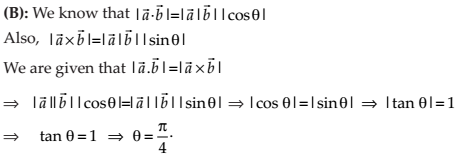error: Content is protected !!